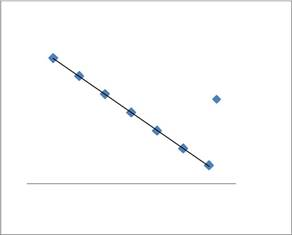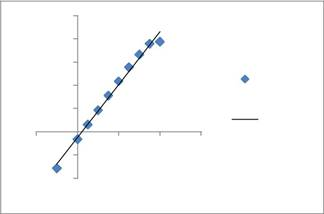Home > mini projects > Small Range Digital Thermometer using 1N4148

Small Range Digital Thermometer simulation on Ngspice using 1N4148 diode as temperature sensor

Abstract - In today's era, we have an ocean of temperature sensing devices available in the industry. In this project we propose a compact and portable design of a small range digital thermometer, using 1N4148 diode as a temperature sensor and Ngspice as a tool for simulation. The temperature coefficient of the diode, -2 mV/Â°C is exploited for this application to create an accurate electronic thermometer. The diode is connected as feedback to an opamp, whose output voltage will be a function of temperature. It will be further amplified by a second opamp, and presented as input to an ADC or voltage scaler. Accordingly, a digital multimeter/display unit can be interfaced to view the reading. The temperature sensitivity and the min/max temperatures are found using Ngspice simulator.

I. INTRODUCTION

THE study of p-n junction characteristics for the design of temperature measurement systems has been done by many researchers. It was established by Ocaya , the

linearity of temperature-voltage relationship for temperature ranges of 0 Â°C to 100 Â°C in a p-n diode, while profiling its voltage, current and temperature behaviour. It was also demonstrated by Godwin  that the 1N4148 diode has linear voltage variations with temperature in the range âˆ’40 Â°C to 150 Â°C. This property is being exploited to make a digital thermometer. The main requirement of today's world is to have an easily portable temperature sensing instrument, fitted with a convenient display unit. Most of the temperature measuring instruments available today are either RTD or thermocouple digital, or thermistor digital thermometers. Most of these require a current sensing device either for calibration, or for measuring purposes. The goal of this project is to propose a device which will combine both these capabilities simultaneously.

In the circuit designed, operational amplifier IC1 741 provides a constant flow of current through the 1N4148 fast signal silicon diode. The variation in temperature causes the drop across the diode to change. This change must be linear, in order to get reliable results. The output voltage of IC1 is thus a function of voltage variation across the diode, which in turn is a function of temperature. This output is amplified by second

operational amplifier IC2. Preset RV1 is used to set the zero-reading on the meter and preset RV2 is used to set the range of temperature measurement.

 Output of ADC Display Our Circuit UnitFig.1. Block Diagram I

The expected output voltage range of IC2 op-amp is 0-5V. This voltage can be given as input to an n-bit ADC which will produce an n-bit digital equivalent corresponding to this voltage. This digital output can be fed into an integrated display unit, or to an LCD / 7-segment display interfaced using embedded systems. [Fig.1.].

 Output of Voltage Multimeter scaler and Our Circuit (mV) shifterFig.2. Block Diagram II

One more flexibility is provided in this model. Rather than using a separate display unit for display, the output voltage can be specifically level-shifted and/or scaled so that numerical value of that scaled voltage in mV is equal to temperature in Â°C. [Fig.2.].

II. CIRCUIT IMPLEMENTATION

Before the hardware implementation of any proposed design, it must first be simulated in software, and checked if it meets our desired specifications or not, whether it gives expected output or not. Based on these results, further course of action will be decided. This project implements the digital thermometer model using Ngspice. Ngspice is a mixed-signal circuit simulator. Spice was originally written at The University of California at Berkeley (USA).To write a Ngspice code, the circuit must first be drawn on project, and all its nodes must be numbered. Then following the syntax as mentioned on Ngspice manual, the components must be included and connected across the nodes. Ngspice supports five types

of analyses viz. transient, dc, ac, transfer and operating point.Fig.3. Circuit for simulation in Ngspice

NGSPICE NETLIST FOR DIGITAL THERMOMETER:

A. Op-amp IC 741 subcircuit:

.SUBCKT 741 1 2 3 4 5

* nodes: 3=+ 2=- 1=out 5=V+ 4=V-

* VCC= 15 VEE= -15 CC= 3e-011 A= 200000 RI= 2e+006

* RO= 75 VOS= 0.001 IOS= 2e-008 IBS= 8e-008

* VSW+= 14 VSW-= -14 CMMR= 90

* ISC= 0.025 SR= 0.5 Fu= 1e+006 Pm= 6.09112e-007

VC 5 15 DC 1.68573V

VE 12 4 DC 1.68573V

IEE 10 4 DC 1.516e-005A

R1 10 0 10Gohm

R6 11 0 100Kohm

R7 5 4 1Kohm

Rc1 6 5 5305.16ohm

Rc2 5 7 5305.16ohm

Re1 9 10 1839.19ohm

Re2 8 10 1839.19ohm

Ro1 1 14 37.5ohm

Ro2 14 0 37.5ohm

Ree 10 0 1.31926e+007ohm

Rcc 0 13 2.20906e-005ohm

Cee 0 10 1e-012

Cc 14 11 3e-011

C1 6 7 1e-016

GA 11 0 6 7 0.000188496

GC 0 13 1 0 45268.1

GB 14 0 11 0 282.942

GCM 0 11 10 0 5.96075e-009

D1 14 13 Dopamp1

D2 13 14 Dopamp1

D3 1 15 Dopamp2

D4 12 1 Dopamp2

Qt1 6 2 9 Qopamp1

Qt2 7 3 8 Qopamp2

.MODEL Dopamp1 D (Is=7.53769e-014A Rs=0 Cjo=0F Vj=750mV Tt=0s M=0)

.MODEL Dopamp2 D (Is=8e-016A Rs=0 Cjo=0F Vj=750mV

Tt=0s M=0)

.MODEL Qopamp1 NPN (Is=8e-016A BF=83.3333 BR=960m

+ Rb=0ohm Re=0ohm Rc=0ohm Cjs=0F Cje=0F Cjc=0F

+ Vje=750m Vjc=750m Tf=0 Tr=0 mje=0 mjc=0 VA=50)

.MODEL Qopamp2 NPN (Is=8.30948e-016A BF=107.143 BR=960m

+ Rb=0ohm Re=0ohm Rc=0ohm Cjs=0F Cje=0F Cjc=0F

+ Vje=750m Vjc=750m Tf=0 Tr=0 mje=0 mjc=0 VA=50)

.ENDS

B. Digital Thermometer:

.include opampmulti.cir

.model ZENER D(BV=4.7 IBV=5e-3 RS=40 IS=1e-12 )

.MODEL 1N4148 d (

+ IS=6.89131e-09 RS=0.636257 N=1.82683 EG=1.15805

+ XTI=0.518861 BV=80 IBV=0.0001 CJO=9.99628e-13

+ VJ=0.942987 M=0.727538 FC=0.5 TT=4.33674e-09

+ KF=0 AF=1 TNOM=27)

X1 2 1 0 10 9 741

X2 6 3 0 10 9 741

V1 9 0 dc 5V

V2 10 0 dc -5V

R1 1 10 100k

D1 2 1 1N4148

R2 3 2 10k

RV2 3 6 100k

R3 3 4 10k

RV1 4 10 100k

dz 0 6 zener

.dc temp -200 350 1

.control

run

display

set color0=white

set color1=black

set xbrushwidth=3

plot v(6)

plot v(2,1)

meas dc output_v find V(2,1) at =-50 meas dc output_v find V(2,1) at =0 meas dc output_v find V(2,1) at =25 meas dc output_v find V(2,1) at =50 meas dc output_v find V(2,1) at =75 meas dc output_v find V(2,1) at =100 meas dc output_v find V(2,1) at =125 meas dc output_v find V(2,1) at =150 meas dc output_v find V(2,1) at =175 meas dc output_v find V(2,1) at =200 .endc

.end

III. RESULTS AND DISCUSSION

The simulation results were recorded and analysed in Ngspice, and the data was further plotted using Microsoft Excel for a better view.

A. Variation of diode drop with Temperature1 V = -0.0025T + 0.4881 RÂ² = 0.9999 diode 0.9 0.8 across 0.7 0.6 Voltage 0.5 Voltage 0.4 0.3 Linear 0.2 (Voltage) 0.1 0 -200 -100 0 100 200

Temperature

Fig.4. Temperature-voltage relationship in the range - 150Â°C to +200Â°C

A linear graph of temperature-voltage relationship was obtained. The equation of the graph was found to be

V = - 0.0025 T + 0.4881, RÂ² = 0.9999

As shown in Fig.4. RÂ² value is very close to unity, showing excellent correlation of the obtained results.

The sensitivity of the diode isThis result is a characteristic of the 1N4148 diode.

B. Variation of Output Voltage with TemperatureVoltage 5 y = 0.0228x - 0.2574 3 4 RÂ² = 0.9896 Output 2 Voltage 1 Linear 0 (Voltage) -100 -1 0 100 200 300

-2 Temperature

Fig.5. Temperature-Output voltge relationship

As the output of first op-amp is passed through a second op-amp in inverting mode, hence the temperature-output voltage relationship is also linear, with a positive slope. The equation of the graph was found to be

V = 0.0228 T - 0.2574, RÂ² = 0.9896

The sensitivity of the device isIV. CONCLUSION

We finally compare the specifications of the device desired, and those met by the simulated device.

Demanded Temperature Range= -50Â°C to 200Â°C Demanded Output Voltage Range= 0V to 5 V

Observed Temperature Range= -57.69 Â°C to 182.69 Â°C Observed Output Voltage Range= -1.75 V to 3.9 V

The observed Results are close to the demanded results. A unique device incorporating temperature sensing, RTD calibrating and current measuring in the specified range can be fabricated as shown in Block Diagram II [Fig.2.]